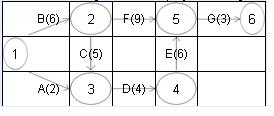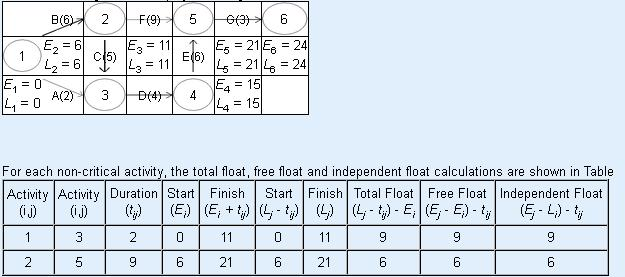# Question & Answer: Your father has appointed you as the project manager for the construction of your new family…

Your father has appointed you as the project manager for the construction of your new family farmhouse. The construction project has seven major activities as listed below. Using the table below, draw the network diagram and derive the earliest completion time TE (or early finish EFT), latest completion time TL(or late finish LFT) and slack for each task. Which tasks are on the critical path? Highlight the critical path on your network diagram. When is the earliest that you can move into the new farmhouse?

 Activity Predecessor ET (wk) TE (EFT) TL (LFT) Slack Critical Path? Permits A – 2 Foundation B – 6 Walls C B 5 Plumbing D A, C 4 Electrical E D 6 Landscaping F B 9 Finishing G E, F 3

Ans:

Don't use plagiarized sources. Get Your Custom Essay on
Question & Answer: Your father has appointed you as the project manager for the construction of your new family…
GET AN ESSAY WRITTEN FOR YOU FROM AS LOW AS \$13/PAGE

The given problem is

 Activity Immediate Predecessors Duration A – 2 B – 6 C B 5 D A,C 4 E D 6 F B 9 G E,F 3

The network diagram for the project, along with activity time, isForward Pass Method
E1=0

E2=E1+t1,2 [t1,2=B=6]=0+6=6

E3=Max{Ei+ti,3}[i=1,2]

=Max{E1+t1,3;E2+t2,3}

=Max{0+2;6+5}

=Max{2;11}

=11

E4=E3+t3,4 [t3,4=D=4]=11+4=15

E5=Max{Ei+ti,5}[i=2,4]

=Max{E2+t2,5;E4+t4,5}

=Max{6+9;15+6}

=Max{15;21}

=21

E6=E5+t5,6 [t5,6=G=3]=21+3=24

Backward Pass Method
L6=E6=24

L5=L6-t5,6 [t5,6=G=3]=24-3=21

L4=L5-t4,5 [t4,5=E=6]=21-6=15

L3=L4-t3,4 [t3,4=D=4]=15-4=11

L2=Min{Lj-t2,j}[j=5,3]

=Min{L5-t2,5;L3-t2,3}

=Min{21-9;11-5}

=Min{12;6}

=6

L1=Min{Lj-t1,j}[j=3,2]

=Min{L3-t1,3;L2-t1,2}

=Min{11-2;6-6}

=Min{9;0}

=0

(b) The critical path in the network diagram has been shown. This has been done by double lines by joining all those events where E-values and L-values are equal.
The critical path of the project is : 1 – 2 – 3 – 4 – 5 – 6
and critical activities are B, C, D, E, G
The total project time is 24
The network diagram for the project, along with E-values and L-values, is